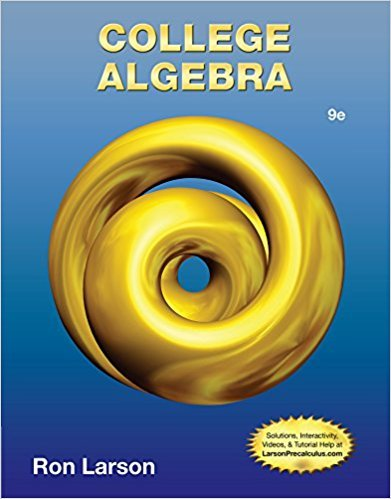×
×

# Solved: Depreciation A hospital purchases a new magneticISBN: 9781133963028 204

## Solution for problem 1.1.75 Chapter 1

College Algebra | 9th Edition

• Textbook Solutions
• 2901 Step-by-step solutions solved by professors and subject experts
• Get 24/7 help from StudySoup virtual teaching assistantsCollege Algebra | 9th Edition

4 5 0 393 Reviews
23
3
Problem 1.1.75

Depreciation A hospital purchases a new magnetic resonance imaging (MRI) machine for \$500,000. The depreciated value (reduced value) after years is given by Sketch the graph of the equation.

Step-by-Step Solution:
Step 1 of 3

\ f . 4"L '\ I I ,J2{ \ ;y t VII {-) -&'/ I -.rdplB \l Uo q/.n\Aler)h.rn fl )-fl".ipat.fi)ta\) q \ Uq-up\Jrn3...

Step 2 of 3

Step 3 of 3

##### ISBN: 9781133963028

College Algebra was written by and is associated to the ISBN: 9781133963028. Since the solution to 1.1.75 from 1 chapter was answered, more than 260 students have viewed the full step-by-step answer. The full step-by-step solution to problem: 1.1.75 from chapter: 1 was answered by , our top Math solution expert on 01/02/18, 09:21PM. The answer to “Depreciation A hospital purchases a new magnetic resonance imaging (MRI) machine for \$500,000. The depreciated value (reduced value) after years is given by Sketch the graph of the equation.” is broken down into a number of easy to follow steps, and 29 words. This textbook survival guide was created for the textbook: College Algebra, edition: 9. This full solution covers the following key subjects: . This expansive textbook survival guide covers 9 chapters, and 5750 solutions.

Unlock Textbook Solution